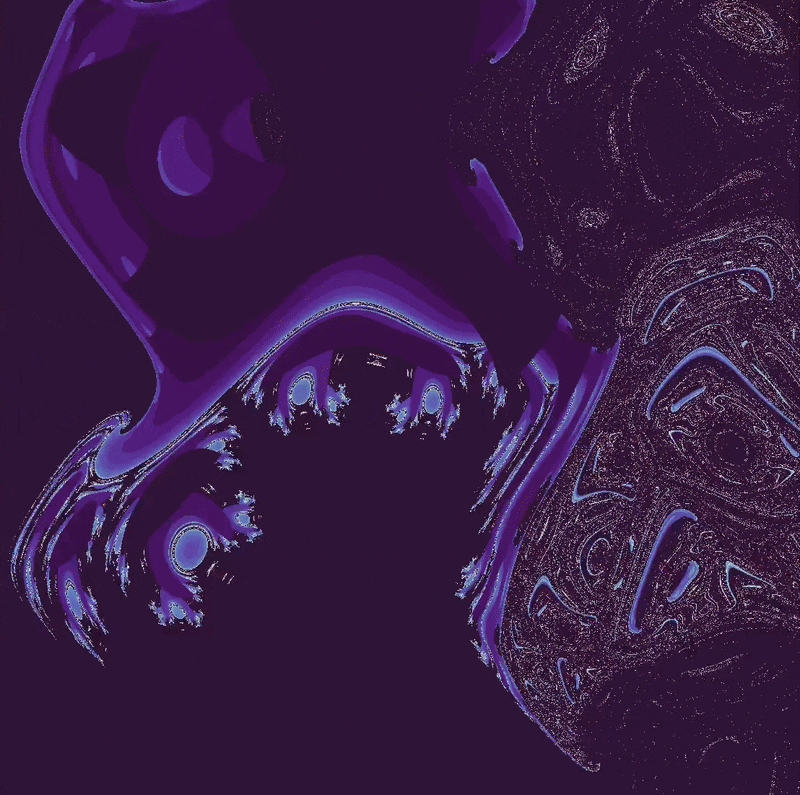## Clifford map boundary

Recall that the Clifford map (see here for more information) is a two dimensional discrete map defined by the following equations:

$x_{n+1} = \sin(ay_n) + c \cdot \cos(ax_n) \\ y_{n+1} = \sin(bx_n) + d \cdot \cos(by_n) \tag{1}$

Regions of space (in this case in the $(x, y)$ plane) that attract all points into a certain attractor are called the basins of attraction (see the henon map page for more information). For example, the attractor of (1) with the starting point $(x_0, y_0) = (10.75, 8.2)$ is also the attractor of the point $(x_0, y_0) = (10.5, 8)$ and the attractor of the point $(x_0, y_0) = (9, 7)$. Other starting points yield other attractors, so does steadily moving the starting point lead to smooth changes between attractors?

For $(x_0, y_0) = (7.5, 7.5) \to (x_0, y_0) \approx (12, 12)$, the transition from one basin of attraction to another is both abrupt and unpredictable: very small changes in starting position lead to total disappearence or change of the attractor for certain values. This causes the attractors to flash when a movie is compiled of $(x_0, y_0) = (7.5, 7.5) \to (x_0, y_0) \approx (12, 12)$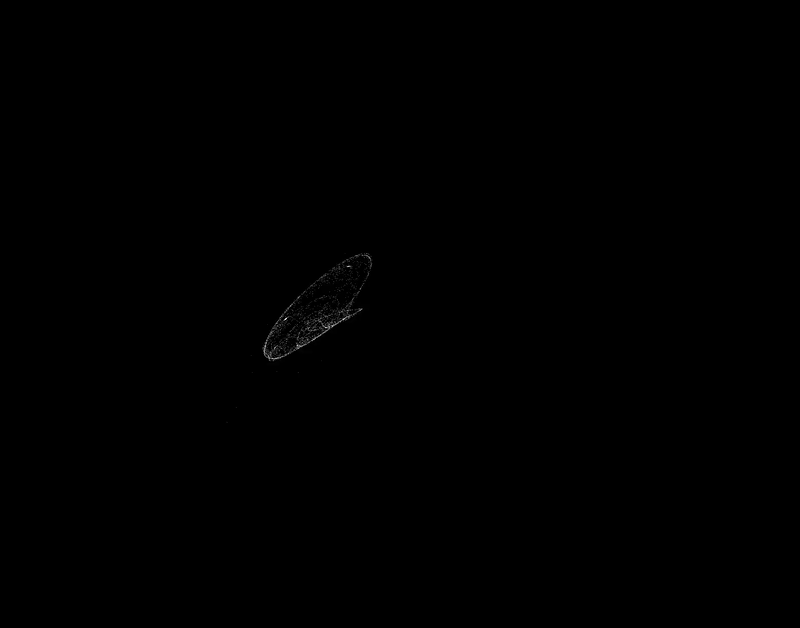### Fractal boundaries for semicontinuous Clifford maps

We can get an idea for the boundaries of a basin of attraction by iterating (2) over a meshgrid, and seeing whether the starting point ends up sufficiently near an attractor, with the assumption that if near enough then future iterations will be stuck at the attractor. This is only an estimate, but seems to provide an accurate portrayal of the behavior of various starting points of the semicontinuous clifford attractor.

For the attractor of the starting point $(x_0, y_0) = (10.75, 8.2)$,

import copy

def clifford_boundary(max_iterations, a=-1.4, b=1.7, c=1.0, d=0.7):
''' A function to map the boundaries of attraction of the
Clifford system, iterated semicontinuously. Takes a number of
maximum iterations and Clifford constants as inputs, outputs
a color-coded map of which values end up in a given basin of
attraction over time.
'''
x_range = 3000
y_range = 3000

x_list = np.arange(8, 12, 4/x_range)
y_list = np.arange(10, 6, -4/y_range)
array = np.meshgrid(x_list, y_list)

x2 = np.zeros(x_range)
y2 = np.zeros(y_range)
iterations_until_in_basin = np.meshgrid(x2, y2)
for i in iterations_until_in_basin:
for j in i:
j += max_iterations

for k in range(max_iterations):
array_copied = copy.deepcopy(array) # copy array to prevent premature modification of x array

# clifford map applied to array
array = array + (1.35*t)*(np.sin(a*array) + c*np.cos(a*array))
array = array + (1.35*t)*(np.sin(b*array_copied) + d*np.cos(b*array))

# note which array elements are enter the basin of attraction
in_basin = np.abs(array - 10.95) + np.abs(array - 8.1) < 1
iterations_until_in_basin[entering_basin] = k

return iterations_until_in_basin


can be called by

plt.imshow(clifford_boundary(30), extent=[8, 12, 6, 10], cmap='twilight_shifted', alpha=1)
plt.axis('on')
plt.show()
plt.close()


which when combined with iterations of (1) starting at $(x_0, y_0) = (10.75, 8.2)$ yields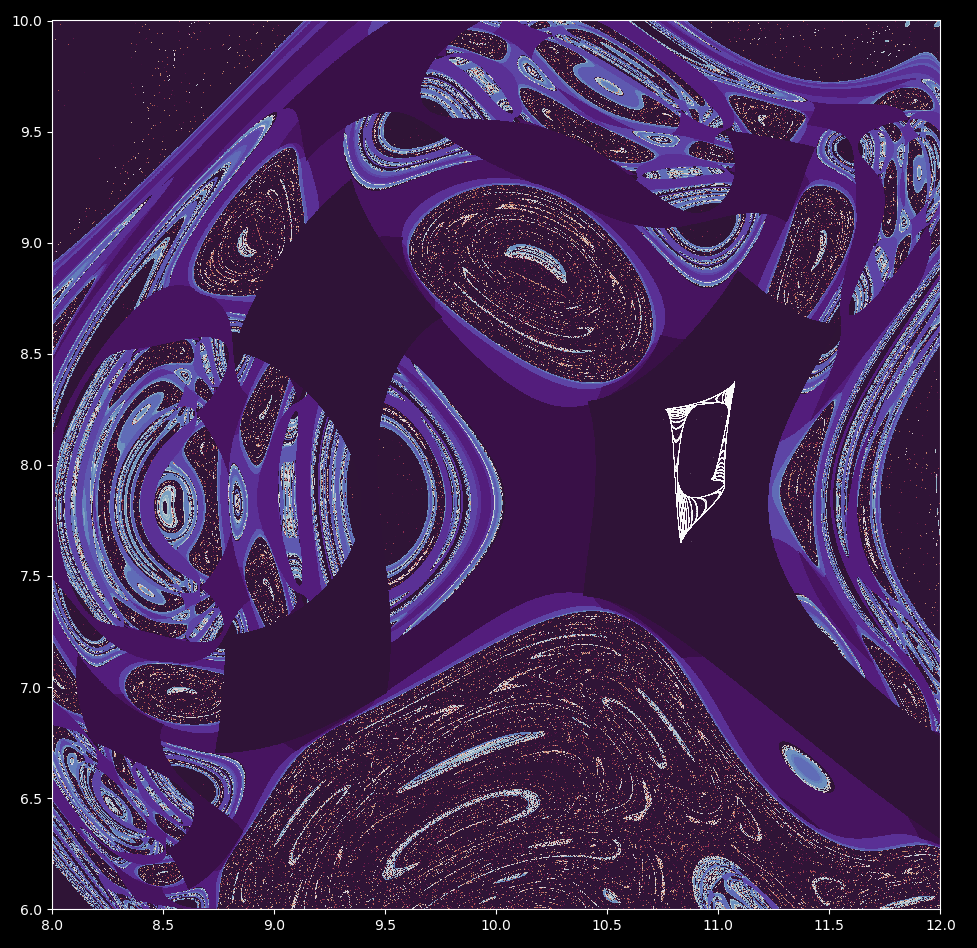The dark area around the attractor is a basin: points are attracted to the region of interest within one iterations. The border of this region are the lighter points, but these form all kinds of fractal patterns such that it is very difficult to tell where exactly the boundary is. With the intricacies of this attractor basin boundary in mind, it is little wonder why slowly moving from one starting point to another causes such drastic changes in which attractor the point heads toward.

This basin seems quite complicated. Is it a self-similar fractal? Lets find out by zooming in on the point $(x, y) = (9.829945, 7.8592)$.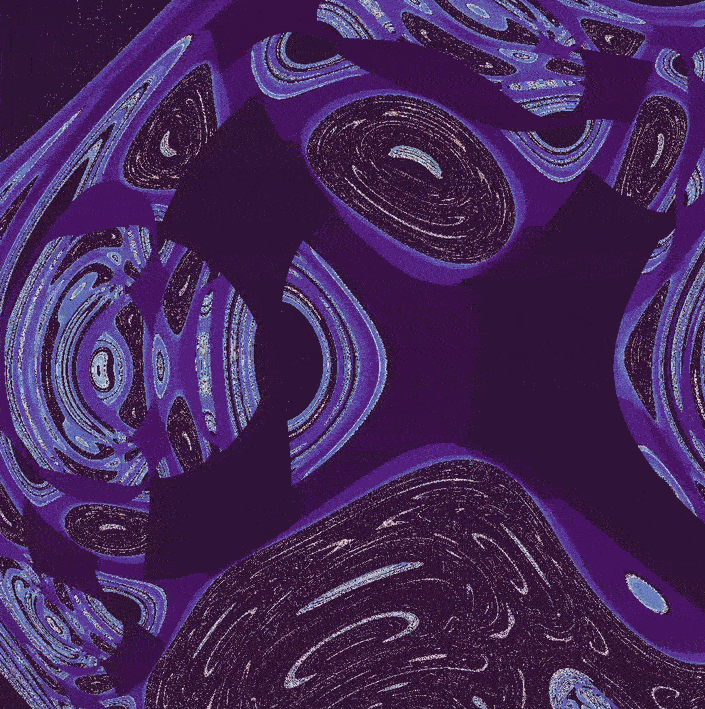There are more and more lines visible as the scale increases. These lines form an irregular Cantor set, a one-dimensional fractal.

So the basin boundary (or at least part of it) is indeed a self-similar fractal. How does this fractal basin boundary form? We can observe what happens when iterating (2) going from $\Delta t=1.05 \to \Delta t=1.35$:What about the attractor for the starting point $x_0, y_0 = 90, 90$? We can see that it too has an extremely intricate boundary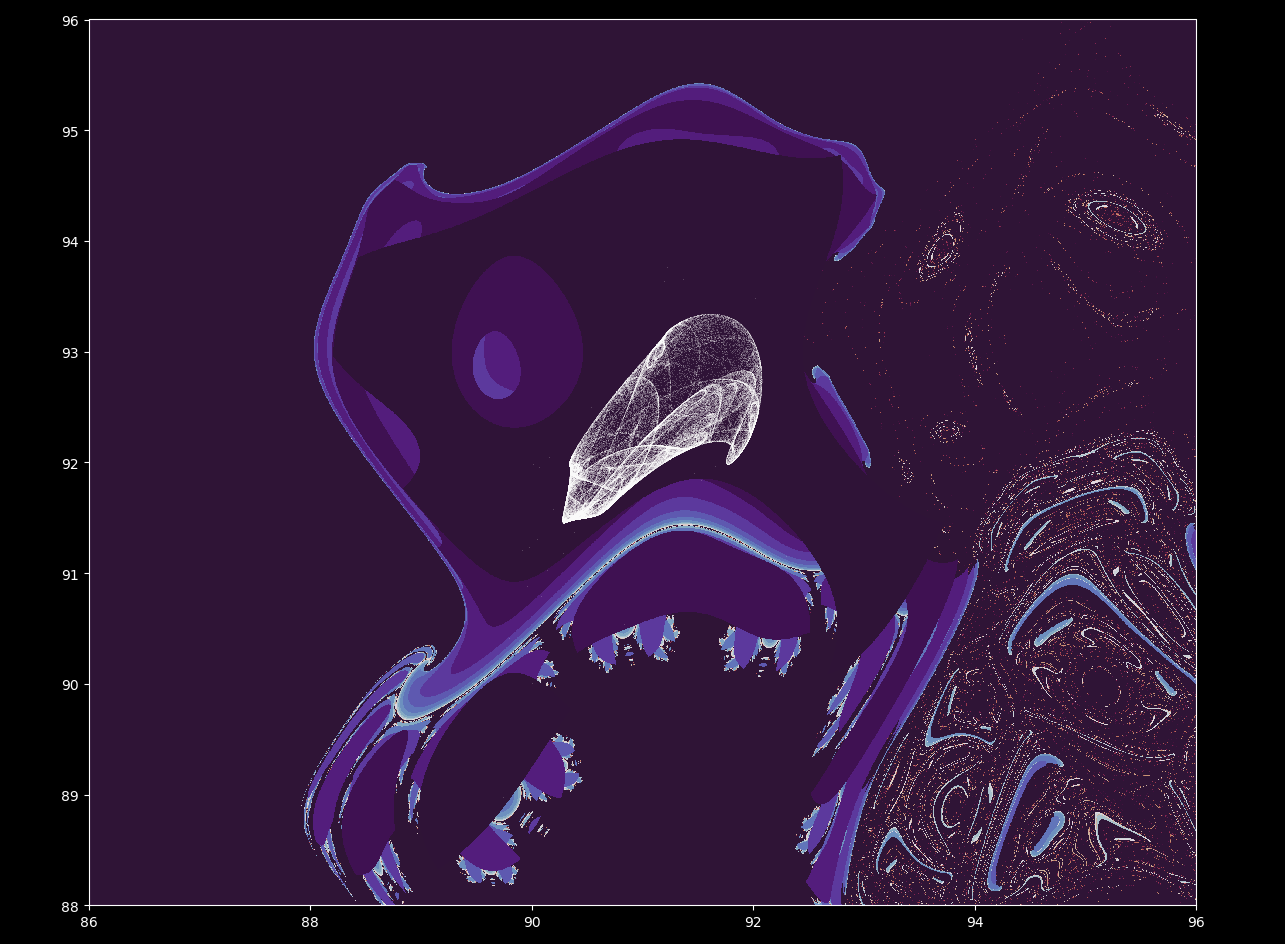and that this boundary transforms from a smooth, relatively simple shape to this more complicated one as $\Delta t=0.5 \to \Delta t=1.5$.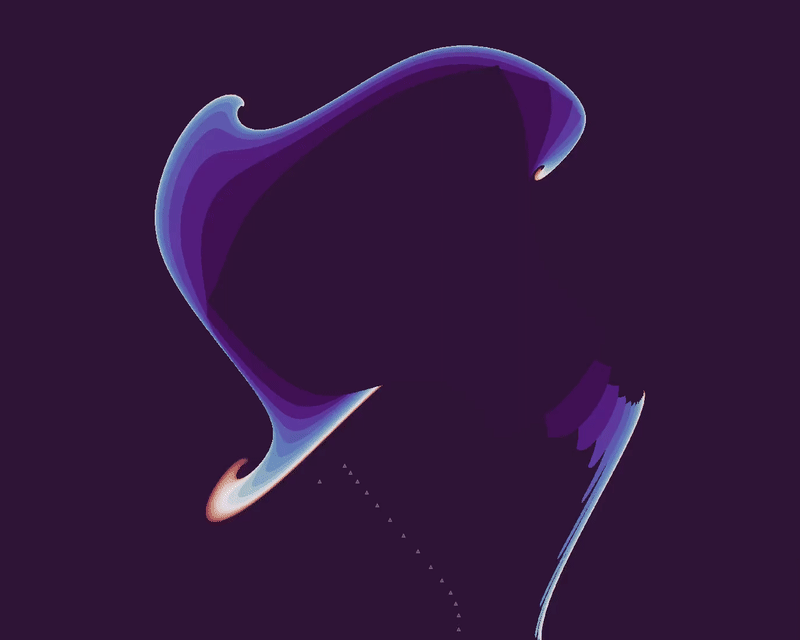Is this boundary a fractal? We can zoom in to find out. Increasing the scale near the point

$(x, y) = (91.82168, 90.47914)$

yields